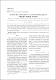## Метрична розмiрнiсть кiстякових дерев унiциклiчних графiв2019
##### Authors
Дуденко, Маргарита
##### Abstract
Найменшу за потужнiстю множину M ∈ V скiнченного графа G = (V,E) таку, що для будьякої пари вершин u,v ∈ V iснує принаймнi одна вершина t ∈ M, для якої має мiсце нерiвнiсть dG(t,v) 6= dG(t,u), називають метричним базисом, а потужнiсть множини M – метричною розмiрнiстю. Оскiльки, як вiдомо, пошук метричної розмiрностi для довiльного графа є NP-важкою проблемою, то пошук метричної розмiрностi графiв обмежують пошуком для певних родин графiв. Для унiциклiчних графiв, тобто графiв, що мiстять рiвно один цикл, пiсля вилучення ребра можна отримати дерево. Метою статтi є встановлення зв’язку мiж унiциклiчним графом, що має метричну розмiрнiсть 2, та метричними розмiрностями його кiстякових дерев залежно вiд способу вилучення ребра
The smallest subset M ⊂ V of a graph G = (V,E) such that for any pair u,v ∈ V there exists at least one vertex t ∈ M with dG(t,v) 6= dG(t,u), is called a metric basis of G, and its cardinality |M| is called the metric dimension of G and denoted by dim(G). Since it is known that ﬁnding the metric dimension of a graph is NP-hard problem, ﬁnding the metric dimension of a graph is usually carried out in certain directions such as description of graph classes for which the metric dimension can be found in polynomial time and calculation of the metric dimension of certain, well-known families of graphs (such as grid graphs, wheels, etc.). One of these areas of research deals with characterization of graph families having a constant metric dimension. In particular, it has been proven that a graph has the metric dimension 1 if and only if it is a path. It is also known that metric dimensions of the cycle Cn, the complete graph Kn and the complete bipartite graph Ks,t are equal to 2, n−1 and s + t−2, respectively. Another area of research on the metric bases of graphs is the ﬁnding the metric dimension of graphs obtained by operations on graphs whose metric bases are known. In this paper, we present the relationship between a unicyclic graph (a graph with exactly one cycle) with metric dimension 2 and the metric dimension of its spanning trees. Since the corresponding spanning trees for a graph are determined ambiguously, depending on the method of deleting the edge to obtain such a tree, its metric dimension may also change. The present paper describes conditions under which a spanning tree of a unicyclic graph G with dim(G) = 2 has the metric dimension from 1 to 4.
##### Keywords
метрика, унiциклiчний граф, метрична розмiрнiсть, метричний базис, кiстякове дерево, вiдстань, стаття, metric, unicyclic graph, metric dimension, metric basis, spanning tree, distance, article
##### Citation
Дуденко М. А. Метрична розмiрнiсть кiстякових дерев унiциклiчних графiв / Маргарита Дуденко // Могилянський математичний журнал. - 2019. - Т. 2. - С. 33-40.